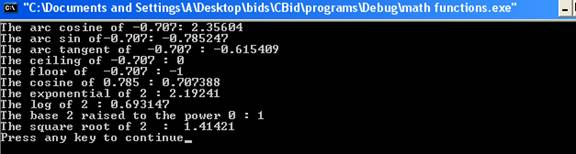Math Functions

The mathematical functions provide the functionality of automatically computing some of the functions such as trigonometric functions, hyperbolic functions, exponential and logarithmic functions and other miscellaneous functions. In order to use these functions header file <cmath> is included into the program. Some of the commonly used functions are:-

• abs() – The function abs() returns the absolute value of integer parameter.

• acos()  -The function acos() returns the arc cosine of the argument. The range of the argument is from -1 to 1. Any argument outside this range will result in error.

• asin()- The function asin() returns the arc sine of the argument. The range of the argument is from -1 to 1. Any argument outside the range will result in error.

• atan()- The function atan() returns the arc tangent of the argument.

• atan2()- The function atan2()  returns the arc tangent of y/x. It accepts two arguments.

• ceil()- The function ceil() returns the smallest integer which is not less than the argument.

• cos() – The function cos() returns the cosine of the argument. The value of the argument must be in radians.

• cosh() –The function cosh() returns the hyperbolic cosine of the argument.

• exp()- The function exp() returns the exponential of the argument.

• fabs()- The function fabs() returns the absolute value of floating point parameter.

•  floor()- The function floor() returns the largest integer which is not greater than the argument.

• fmod()- The function fmod() returns the remainder of floating point division of the two arguments.

• log() – The function log() returns the natural logarithm of the argument. An error is encountered if the argument is negative or zero.

• log10()- The function log10() returns base 10 logarithm of the argument. An error occurs if the argument is negative or zero.

• pow()- The function pow() returns the base raised to the power.

• sin()- The function sin() returns the sine of the argument. The value of the argument must be in radians.

•  sinh()- The function sinh() returns hyperbolic sine of the argument.

• sqrt()- The function sqrt() the square root of the argument. An error occurs if the value of the argument is negative.

•   tan()- The function tan() returns the tangent of the argument. The value of the argument must be in radians.

• tanh()- The function returns the hyperbolic tangent of the argument.

Here is a program which illustrates the working of the math functions.

#include<iostream>

#include<cmath>

using namespace std;

int main()

{

double a=-0.707;

double a1=(45.0*3.14)/180;

double a2=2;

double b=0.0;

b=acos(a);

cout << "The arc cosine of " << a << ": " << b << endl;

b=asin(a);

cout << "The arc sin of" << a << ": " << b << endl;

b=atan(a);

cout << "The arc tangent of  " << a << " : " << b << endl;

b=ceil(a);

cout << "The ceiling of " << a << " : " << b << endl;

cout << "The floor of  " << a << " : " << floor(a) << endl;

cout << "The cosine of " << a1 << " : " << cos(a1) << endl;

cout << "The exponential of " << a2 << " : " << exp(a1) << endl;

cout << "The log of " << a2 << " : " << log(a2) << endl;

cout << "The base " << a2 << " raised to the power " << b << " : "  << pow(a2,b) << endl;

cout << "The square root of " << a2 << "  :  " << sqrt(a2) << endl;

return(0);

}

The result of the program is:-The statement

#include<cmath>

includes a header file <cmath> into the file. The statement

b=acos(a);

returns the arc cosine of argument a. The arc cosine of -0.707 is 2.35604. The statement

b=asin(a);

returns the arc sine of argument  a. The arc sin of a -0.707 is -0.785247. The statement

b=atan(a);

returns the arc tangent of argument a. The arc tangent of -0.707 is -0.615409. The statement

b=ceil(a);

returns the ceiling of argument a. The ceiling of -0.707 is 0. The statement

cout << "The floor of  " << a << " : " << floor(a) << endl;

displays the floor of argument a. The floor of -0.707 is -1. The statement

cout << "The cosine of " << a1 << " : " << cos(a1) << endl;

displays the cosine of the argument a1. The cosine of 45 degrees is 0.707. The argument a1 contains the radian value of 45 degrees. The statement

cout << "The exponential of " << a2 << " : " << exp(a1) << endl;

displays the exponential of argument of a2. The exponential of 2 is 2.19241. The statement

cout << "The log of " << a2 << " : " << log(a2) << endl;

displays the logarithm of argument a2. The logarithm of 2.0 is 0.69. The statement

cout << "The base " << a2 << " raised to the power " << b << " : "  << pow(a2,b);

displays the base a2 raised to the argument b. The base 2.0 raised to the power 0 is 1. The statement

cout << "The square root of " << a2 << "  :  " << sqrt(a2) << endl;

displays the square root of the argument a2. The square root of 2.0 is 1.414.

Go to the previous lesson or proceed to the next lesson